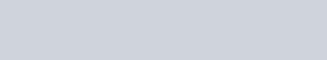Livio/ May 19, 2019/ Python/ 0 comments

Naive Bayes Classifier

The Naive Bayes classifier is a simple algorithm which allows us, by using the probabilities of each attribute within each class, to make predictions. It makes the strong assumption that the attributes within each class are independent, which means that attribute x happening does not influence the probability of y happening. In spite of this strong assumption, Naive Bayes has worked very well in many complex scenarios.

Bayes Theorem

The Naive Bayes algorithm is built upon the Bayes’ theorem which states that the probability of event A occurring given that event B occurs is equal to the probability of event B occurring given that event A occurs times the probability of event A occurring, divided by the probability of event B occurring:Building a spam filter

Naive Bayes can be used to build a spam filter from scratch. The aim will be, given the words contained in a message, to determine the probability of that message being spam or not. Our equation will be:The left part of the equation translates to the probability of the message being spam (y) given the words x1, x2, …., xn. The right part of the equation translates to the probability of a message being spam P(y), times the probability that a spam message contains the words x1, x2, x3, …, xn, divided by the probability that a message (no matter whether it’s spam or not) contains the words x1, x2, x3, …, xn. Given that the attributes (occurrence of the words) are assumed to be independent as stated earlier, the equation becomes:The product of the probabilities of each word occurring can also be written as:in order to avoid multiplying many probabilities (0 to 1 numbers) together and generating possible underflow.

The data

In this post we will use the data which I found at this link. It contains a text file where each line represents a message. If the line begins with ham then this wasn’t a spam message, whereas if it begins with spam then this was a spam message:Building the Naive Bayes class

The first method we need to add to our class is a function to extract single words from a message. We can use a simple regular expressions pattern to do this:

We will load the data to our class as a list of tuples, where the first element of the tuple tells us where the message is spam or not and the second item represents the message itself. So we will need a function which, for each message, keeps track of the number of spam messages, the number of non spam message and the number of occurrences of each word within spam message and within non spam messages.

and now we just need to add the last method which tells us the probability of a message being spam:

Testing the model

To test our model, we will first create a simple function which will split the data into a training set and a testing set:

after testing the model a few times, we can see it outputs a very high precision: